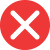20st

Incorrect spelling, explanation: since st is the ending for ordinal numbers with first at the end (for example 31st, 101st), and th is the ending for all the numbers with it as the final sound, 20st is the wrong form. Following the mentioned rule, the correct form is 20th, as in twentieth. Thus 20th is the correct form.20th

Correct spelling, explanation: 20th is the correct way to write this number because th is the sound present at the end of the full-word form twentieth. St is the ending for ordinal numbers with such sound at the end, therefore 20st is incorrect. 20th is the only correct form.

Definition of 20th:
numeral, the ordinal number between 19th and 21st
20th Century Fox has produced many blockbuster films.
This is the 20th day we are waiting for test results, this is ridiculous!20nd

Incorrect spelling20rd

Incorrect spelling